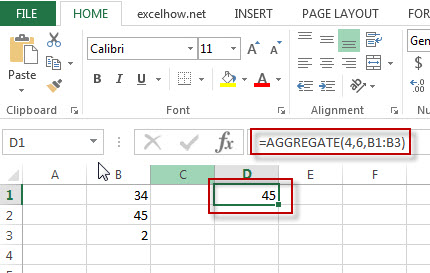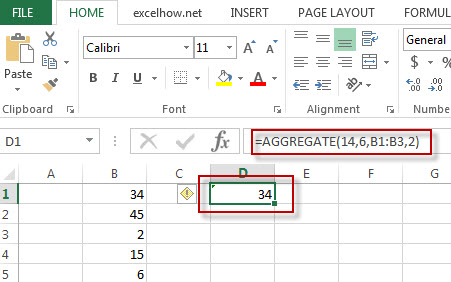# Excel AGGREGATE Function

This post will guide you how to use Excel AGGREGATE function with syntax and examples in Microsoft excel.

### Description

The Excel AGGREGATE function returns an aggregate in a list or database and ignore errors or hidden rows.it allow you to apply functions such as: SUM, COUNT, MAX, MIN, SMALL and etc.

The AGGREGATE function is a build-in function in Microsoft Excel and it is categorized as a Math and Trigonometry Function.

The AGGREGATE function is available in Excel 2016, Excel 2013, Excel 2010, Excel 2007, Excel 2003, Excel XP, Excel 2000, Excel 2011 for Mac.

### Syntax

The syntax of the AGGREGATE function is as below:

= AGGREGATE(function_num, options, ref1,[ref2])

Where the AGGREGATE function arguments are:
function_num – This is a required argument. The function that you want to use and it can be any of the below number(1-19).

 1 AVERAGE 2 COUNT 3 COUNTA 4 MAX 5 MIN 6 PRODUCT 7 STDEV.S 8 STDEV.P 9 SUM 10 VAR.S 11 VAR.P 12 MEDIAN 13 MODE.SNGL 14 LARGE 15 SMALL 16 PERCENTILE.INC 17 QUARTILE.INC 18 PERCENTILE.EXC 19 QUARTILE.EXC

Options – This is a required argument.  A numeric value that determines which values to ignore. If the options is omitted,  the options value will be set to 0.

 Value Explanation 0 Ignore nested SUBTOTAL and AGGREGATE functions 1 Ignore nested SUBTOTAL, AGGREGATE functions, and hidden rows 2 Ignore nested SUBTOTAL, AGGREGATE functions, and error values 3 Ignore nested SUBTOTAL, AGGREGATE functions, hidden rows, and error values 4 Ignore nothing 5 Ignore hidden rows 6 Ignore error values 7 Ignore hidden rows and error values

Ref1 – This is a required argument. The first numeric argument for the functions in aggregate function.
Ref2 – This is an optional argument. Numeric arguments 2 through 253.

### Example

The below example will show you how to use Excel AGGREGATE function to return an aggregate in a list.

#1 = AGGREGATE (4,6,B1:B3)Note: the above formula will calculate the maximum value in the range B1:B3 and ignoring error values.

#2 = AGGREGATE (14,6,B1:B3,2)Note: The above excel formula will calculate the second largest value in the range B1:B3 and ignoring error values.